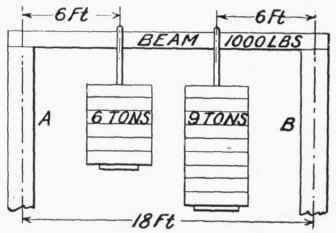Since a strain is the lengthening due to the action of a stress it is measured in fractions or decimals of an inch.

To illustrate: If a bar of steel, such as a piston rod, has been stretched or lengthened 1/64 in. by the stress caused by the weight of the driving box, the strain in the steel rod is 1/64 in.

If a weight is hung from a beam resting on two supports A and B as in Fig. 100, the beam is a lever of the second class. If we consider the pressure on the support A as the power and the pressure on the support B as the fulcrum, we can easily find the power if we know the weight. Then knowing the power, we can find the pressure on support B, provided we know the distance of the weight from one end and the distance between the supports. It makes a difference which support we consider to be the fulcrum.

If the weight were hung from the center of the beam, it is plain that each support would carry one-half of the load. But if, as shown in the figure, the weight is hung a distance equal to one-fourth the length of the beam from A, the support A will bear three-fourths of the weight and the support B will bear one-fourth.

Example. - Suppose instead of one weight, we had two weights hanging on a 1000-lb. steel beam as shown in Fig. 101. What will be the pressure or weight on supports A and B? Consider one end of the beam, A, as the fulcrum. Then the moment of the 0-ton weight will be: 6 X 6 or 36, and the moment of the 9-ton weight will be: 9 X 12 or "108. The moment of W, the weight supported at B, will be 18 X W. Then since the sum of the moments of the weights will be equal to the moment of the weight supported at B, we will have: 36 + 108 = 18 X W, or W = 8 tons, for the weight supported at B. But the beam itself weighs 1000 lbs., one-half of which is supported at A and the other half at B. Adding this to W makes 8 tons plus 1/4 ton, or 8 1/4 tons for the total weight supported at B. The weight supported at A will, of course, be the amount left after subtracting the weight at B from the total weight:

6 tons + 9 tons + 1/2 ton = 15 1/2 tons, total weight and 15 1/2 tons - 8 1/4 tons = 7 1/4 tons, the weight supported at AFIG. 100.Fig. 101.